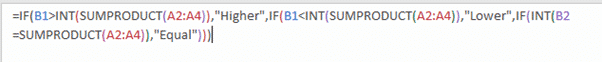# LET() assigns names to calculations in Excel

Let() is a new function coming to Excel 365 which will be popular with makers of long, complicated functions.

The new function lets you name a temporary variable to use within a formula.  That might seem a strange thing to do but it’s a godsend for advanced Excel formulas.

Longer Excel formulas often have repetition, the same calculation is done many times. Or, in other words, the same sub-formula repeats.

For example, nested IF statements usually have the same test repeated many times.

`=IF(B1>INT(SUMPRODUCT(A2:A4)),"Higher",IF(B1<INT(SUMPRODUCT(A2:A4)),"Lower",IF(INT(B2=SUMPRODUCT(A2:A4)),"Equal")))`Has the calculation INT(SUMPRODUCT(A2:A4)) done three times.

The repetition makes the whole thing harder to read and easier to mess up.  Accidentally changing one of the sub-calculations and not the others.

Repeating formula strings have to be separately processed by Excel which is a waste of computer resources.  That’s a minor issue in the above example but imagine a big worksheet with hundreds or thousands of similar statements, all being individually calculated.

## Introducing LET()

LET fixes those problems by allowing you to calculate a formula once, give it a name, then use that name many times in the cell formula.

In it’s simple form, LET() looks like this:

`LET(<variable name>,<value or calc for the variable>,<main formula for the cell>)`

a very simple example could look like this:

`LET(pi, 22/7,pi*(Radius^2))`

You can add more pairs of variable & value like this:

`=LET(pi, 355/113,e,2.718282,pi*(Radius^e))`

(these are just examples, in practice you’d use PI() and EXP(1) for those values).

Microsoft has a little graphic to explainSource: Microsoft

LET can have up to 126 pairs of name and variable.

Here’s the same formula that we started with, this time using LET()

The calculation INT(SUMPRODUCT(A2:A4)), is only done once and named iX.

`=LET(iX,INT(SUMPRODUCT(A2:A4)),IF(B1>iX,"Higher",IF(B1<iX,"Lower",IF(B1=iX,"Equal"))))`Changing the test now needs only a single change. Excel only evaluates ‘ix’ once instead of three times.

## What’s new?

Hang on, I hear you cry?  We can do LET already by putting the calculations in another cell, giving that cell a name then using the name in the calculation.

That’s true, our example could be done like this:

• Enter the formula =INT(SUMPRODUCT(A2:A4)) into a cell.
• Name that cell ‘iX’
• In the main cell use a formula that references the named cell: =IF(B1>iX,”Higher”,IF(B1<iX,”Lower”,IF(B1=iX,”Equal”))))

That gives you the same result by a similar method.  It has the advantage of working for multiple cells and in earlier versions of Excel.

However, it’s less efficient than using LET() within a single cell.

LET is also self-contained and readable without reference to other cells.

Generally speaking, if the same calculation is being done within many cells, it’s better done once in a single named cell.

Calculations repeated within a single cell can use LET().

LET() is currently only available for Fast track Insiders with Excel 365 for Windows.  It’ll gradually be rolled out to other Insiders and eventually public releases.

IF and Nested IF Statements in Excel

More Excel alternatives to nested IF statements

SumIF, a better way to Sum in Excel

Join Office for Mere Mortals today

Office for Mere Mortals is where thousands pick up useful tips and tricks for Word, Excel, PowerPoint and Outlook.

Give it a try. You can unsubscribe at any time.  We've never spammed or sold addresses since we started over twenty years ago.